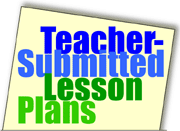Home >> A Tsl >> Archives >> 04 1 >> Fast Facts

## Search formFast Facts

Subjects

• Arithmetic

• K-5

Brief Description

This game offers a fun, hands-on way to reinforce basic math-facts skills.

Objectives

Students
• practice/ reinforce basic math facts.

Keywords

addition, multiplication, subtraction, facts, game, dice

• dice (at least one per student)
• pencil
• paper
• timer

The Lesson

Before the lesson:
Divide the class into 2 teams. Arrange each team in a circle, one circle inside the other. Each student in the inside circle should face a student in the outside circle. Students can be seated at desks but this activity is probably best done on the floor. Note: The students on the inside circle will not move as the game proceeds.

Every student should have a sheet of paper, a pencil, and a single die. Have the students write their names in the top left corner of the paper.

Decide in advance whether you will use the game to reinforce students' addition or multiplication fact skills.

The Lesson
Share the rules of the game:

• You might begin with one simple rule: "Use your quiet voices when you answer so you do not disturb others around you."
• The two students in each facing pair will roll his/her dice at the same time.
• As soon as the dice stop rolling, students perform the assigned operation on the dice. The first student to call out the correct answer earns a point.
For example, if the operation is multiplication and one student rolled a 6 and the other rolled a 4, the first student in the pair to call out 24 (6 x 4 = 24) earns the point.
• Students continue doing this for 2 minutes. At the end of two minutes, the students in the outside circle only rotate clockwise one person so they now have a different partner.
• At the end of the allotted time, have students total their points. Provide a prize to the student with the most correct answers.

Variations

• You can use this game review subtraction or to review multiplication of one- and two-digit numbers. Give students more than 1 dice.
For example, if one student rolls a 6 and a 3 (18) and another rolls a 5 and a 2 (10), then 18 - 10 = 8 or 18 x 10 = 180.
• You can teach commutative or associative properties of addition/multiplication. For example, the identified operations might be subtraction and multiplication. The first student rolls a 5 and the second student rolls a 2 (5 - 2); then one of the students rolls another die to reveal a 5. The resulting equation is (5 - 2) X 5 = 15.
• Instead of dice, you can use playing cards to as a toll to help students review math facts 1 to 10.

Assessment

Students might record all of the problems on their papers and the papers might be checked for a grade.

Submitted By

Linda Nation, Crockett Elementary School in Bryan, Texas

Education WorldÂ®